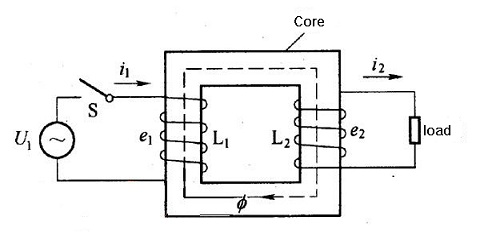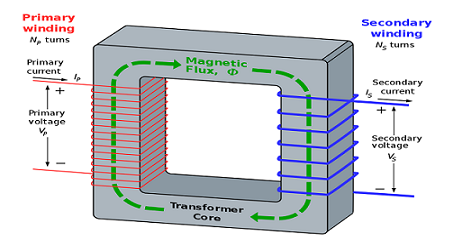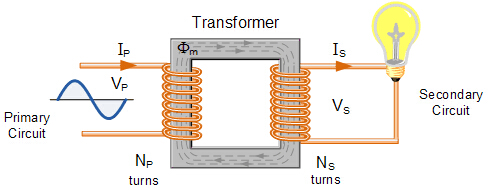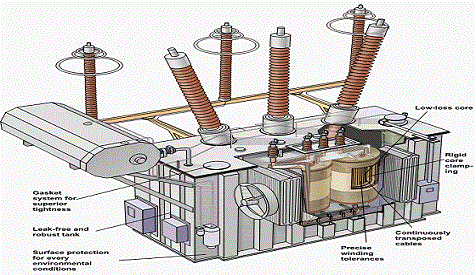HomeBlog

# What is Transformer？ Transformer Basics and Transformer Principles

Transformer is an essential part in electrical equipment. So it is necessary to know and master the basic knowledge of it. Is a necessary skill of every electric design.

Catalogue

 Article Core Transformer Common Sense DefinitionOperationClassificationComponentsFunctionAutotransformerVoltage RegulationRelation of CoilsVoltage Change RateRated Voltage OutputSmall TransformerRunning LossesSpecificationSelectionOverload Operation Test

This video gives a detailed animated illustration on the working of electrical Transformers. Here the basic working principle and construction of transformer, step-up transformer, step-down transformer, transformer winding and core construction are well illustrated.

What is transformer?

Transformer is a device that uses the principle of electromagnetic induction to change the AC voltage. The main components are primary coil, secondary coil and core (magnetic core). The main functions are: voltage conversion, current conversion, impedance transformation, isolation, voltage stabilization (magnetic saturation transformer) and so on. It can be divided into power transformer and special transformer (furnace transformer, rectifier transformer, power frequency test transformer, voltage regulator, mine transformer, audio transformer, intermediate frequency transformer, high frequency transformer, impulse transformer, instrument transformer, electronic transformers, reactors, voltage and current transformer, etc.)The role of the core is to strengthen the magnetic coupling between the two coils. In order to reduce the eddy current and hysteresis loss in the iron, the iron core is formed by the superposition of the painted silicon steel sheet; there is no electrical connection between the two coils, and the colis are wound by insulated copper wire (or aluminum wire). One coil connected to the AC power supply is called the primary coil (or the primary coil) and the other coil is the secondary coil connected electrical appliances. The actual transformers are very complicated, so there may be problems exist to concern, such as copper loss (coil resistance heating), iron loss (core heating), magnetic flux leakage (air-closed magnetic induction line) and so on.

To simplify the discussion, an ideal transformer is introduced. Ideal transformer requires some necessary conditions: ignoring the flux leakage, ignoring the resistance of the primary and secondary coils, ignoring the loss of the iron core, and ignoring the no-load current (the current in the primary coil which supply the secondary coil). For example, the power transformer is close to the ideal condition when it is running at full load (the output with rated power of the secondary coil).

Transformer is a static electrical appliance made by the principle of electromagnetic induction. When the primary coil of the transformer is connected to the AC power supply, the core produces an alternating flux, which is represented by φ. The φ in the primary and secondary coil is the same, and φ is also a simple harmonic function, and φ = φ msinωt. According to Faraday's law of electromagnetic induction, the induction electromotive force in the primary and secondary coils is e1=-N1d φ/dt, e2=-N2d φ /dt. N1, N2 is the number of turns of the secondary coil. From the diagram, we can see that U1=-e1, U2=e2(the primary coil physical quantity is represented by the subscript 1, the secondary coil physical quantity is indicated by the subscript 2), and the complex-effective value is U1=-E1=jN1 ω Φ, U2=E2=-jN2 ω Φ, and makes transformer ratio k=N 1 /N 2. From the upper formula, we can get U1 /U2=-N1 /N2=-k. that is, the voltage effective value of the transformer to that of two coils, which is equal to its coil-voltage ratio, and the phase difference of the voltage of two coils is π.

Further More Based On Above Mentioned

U1/U2=N1/N2

Under the condition that the no-load current can be neglected, there is I1 /I2=-N2 /N1, that is, the effective value of the coils current is inversely proportional to the number of turns, and the phase difference is π.

On the contrary, under the condition of no-load current,

I1/ I2=N2/N1

The power of the ideal transformer is equal to that of the subcoils, that is P1=P2. It shows that the ideal transformer itself has no power loss. But there is always a loss in the actual transformer, and its efficiency is η= P2 /P1, for example, although power transformer efficiency is very high, can reach over 90%, still has a little loss.

In AC circuit, the equipment that increases or decreases the voltage is called transformer. The transformer can transform any voltage into the value we need at the same frequency to meet the requirements of transmission and distribution. For example, the power generated by a power plant has a lower voltage level, which must be increased the voltage to transmit to a far distance, and the power area must reduce the voltage to a suitable voltage level for power equipment and daily use.

2. How transformer works?

Transformers are based on electromagnetic induction. It consists of an iron core made of silicon steel sheet (or silicon steel sheet) and two sets of coils around the core. The core and the coil are insulated from each other without any electrical connection. The coils connected to one side of the transformer and the power supply are called primary coils (or primary sides), and the coils connect transformers and electrical equipment are called secondary coils (or secondary sides).

When the primary coil of the transformer is connected to the AC power supply, the changing magnetic field line in the core appears. Because the secondary coil is wound on the same iron core, the magnetic field line cuts the secondary coil, and the inductive electromotive force must be generated on the secondary coil, finally the voltage at both ends of the coil generated. Because the magnetic line is alternating, the voltage of the secondary coil is also alternating. And its frequency is exactly the same as the frequency of the power supply. It is proved by theory that the voltage ratio between the primary coil and the secondary coil is related to the turns of coils. It can be expressed as follows:

Primary coil voltage / secondary coil voltage = primary coil turns / secondary coil turns, the higher the number of turns, the higher the voltage. Therefore, it can be seen that the turns of secondary coil is less than primary coils, that is, step-down transformer, otherwise, it is a step-up transformer.3. What types of transformer are there?

According to the number of phase, there are single-phase and three-phase transformers; according to the function, there are power transformers, special power transformers, voltage regulating transformers, measuring transformers (voltage transformers, current transformers), small power transformers (for small power equipment), safety transformers; according to the structure, there are core type and shell type; according to the coil, there has double winding and multi-winding transformers, auto-transformer; according to the cooling mode, oil-immersed type and air-cooled type transformers.4. What are the components of the transformer?

Transformer components are mainly composed of iron core, coil, also have other parts, such as oil tank, oil pillow, insulating sleeve and splice, etc.5. What’s the function of transformer oil?

The functions of transformer oil are:

(1) insulation;

(2) heat dissipation;

(3) elimination of arc.

6. What is autotransformer?

The autotransformer has only one set of coils, and the secondary coils are tapped from the primary coils, and its electricity can transmitted. It not only has electromagnetic induction, but also the transmission of electricity. There are fewer silicon steel sheets and fewer copper wires in this kind of transformer than in ordinary transformers, often used to voltage regulator.

7. How voltage regulator works?

The voltage regulator is constructed the same as the autotransformer, but the iron core is made into a ring coil. The secondary coil tap uses a sliding brush contact to make the surface of the ring along the contact slip in a circular way to achieve voltage regulation smoothly.

8. What is the current relationship between the primary coil and the secondary coil of the transformer?

When the transformer operates with load, the current change of secondary coil will cause the corresponding change of primary coil current. According to the principle of magnetic potential balance, it is deduced that the current of the primary and secondary coil is inversely proportional to the number of turns of the coil, the current is small with more turns, and the current with less turns is large. The following formula can be expressed: primary coil current / secondary coil current = secondary coil turns / primary coil turns.

9.What is the voltage change rate of a transformer?

Voltage change rate of voltage regulator is one of the main indexes of transformer performance. When the transformer supplies power to the load, the voltage at the load end of the transformer will inevitably decrease. Comparing the reduced voltage value with the rated voltage value, the percentage is the rate of voltage change. It can be expressed by the formula: voltage change rate = [(secondary rated voltage-load terminal voltage) / secondary rated voltage] ×100%. Generally, for the normal power transformer, when connected to the rated load, the voltage change rate is 4% to 6%.

10. How to ensure that the transformer has a rated voltage output?

Too high or too low voltage will affect the normal operation and service life of the transformer, so the voltage must be adjusted. The method of voltage regulation is to draw out several taps in the primary coil and connect them to the tap beginning, which changes the number of turns of the coil by turning the contact. In addition, the required rated voltage can be obtained by rotating the position of the tap switch. It also needs to note that voltage regulation usually occurs after the load of the transformer is cut off.

11. What kind of small transformers are usually used? Where are they applied?

Small transformers refer to single-phase transformers with a capacity below 1k VA, mostly used as power transformers for electrical equipment control, electronic equipment and safe lighting equipment.

12.What are the losses of transformers in operation? How to reduce them?

The loss of transformer in operation includes two parts.

(1) One is caused by the iron core. When the coils are electrified, the magnetic field lines are alternating and cause eddy current and hysteresis loss in the core.

(2) Another loss is caused by the resistance of the coil itself. When the primary and secondary coils of the transformer have current passing through, some electrical energy may loss.

The sum of iron loss and copper loss is the transformer loss, which is related to transformer capacity, voltage and equipment utilization. Therefore, in the selection of transformers, the capacity of the equipment and the actual usage should be as consistent as possible, in order to improve the utilization rate of equipment, pay attention not to make the transformer lies in light load operation.13. What is the nameplate of the transformer? What are the main technical data on the nameplate?

The nameplate of the transformer should indicate the transformer's performance, technical specifications and use occasions to meet the needs of the user. The main technical data usually selected are as follows:

(1) The number of rated capacity. The output capacity of the transformer is rated. For example, the rated capacity of single phase transformer is Uline × I line, and the capacity of three phase transformer is also the U line × I line.

(2) Rated voltage volts. Indicate the terminal voltage of the primary coil and the secondary coil (when the load is not attached). Note that the terminal voltage of the three-phase transformer refers to the line voltage U-line value.

(3) Rated current amperes. It means LineI current value that allows long-term passage of primary and secondary coils at rated capacity and allowable temperature rise.

(4) Voltage ratio. It is the ratio between primary coil rated voltage and secondary coil rated voltage.

(5) Line connection mode. Single-phase transformers have only a set of coils of high and low voltage, only for single-phase use, and three-phase transformers have Y/△type. In addition to the above technical data, there are transformer rated frequency, phase number, temperature rise, impedance percentage of transformer, etc.14. How to choose a transformer? How to determine the reasonable capacity of transformer?

First of all, it is necessary to investigate the power supply voltage of the place where the electricity is used, the actual power load of the user and the conditions of the place where it is located, and then select one by one according to the technical data indicated by the nameplate of the transformer, generally from the capacity and voltage of the transformer. Considering the current and environmental conditions, the capacity selection should be based on the capacity, nature and service time of the user's power equipment to determine the required load, and then select the transformer capacity.

In normal operation, the power load of transformer should be about 75% ~ 90% of the rated capacity of transformer. When the actual load of the transformer is less than 50%, the small capacity transformer should be used, and the large transformer should be replaced immediately if the rated capacity of the transformer is greater than that of the transformer. At the same time, when selecting the transformer to determine the primary coil voltage of the transformer according to the line power supply and the voltage value of the secondary coil according to the electrical equipment, it is best to select the low-voltage three-phase four-wire power supply system. This can provide power supply foe entire operation.

For the selection of current, attention should be paid to that the load can meet the requirements of the motor when it starts (because the starting current of the motor is 4 ~ 7 times larger than that of the sinking operation).

15. Why transformer can’t run when overload?

Overload operation refers to the transformer operating in excess of the current specified on the nameplate. Overload is divided into normal overload and accident overload. The former refers to the increase of power consumption under normal power supply, and it often makes transformer temperature rise, impels transformer insulation to aging, and reduces service life. Therefore, transformer overload is not allowed. In special cases, the overloading of transformers in a short period of time should not exceed 30% of the rated load in winter, and not more than 15% in summer. For the latter, the accident overload and allowable time requirements are as follows:

 Multiple of Rated Load Reasonable Time of Overload Indoors Outdoors 1.30 2 hours 1 hour 1.60 30 minutes 15 minutes 1.75 15 minutes 8 minutes 2.00 7.5 minutes 4 minutes

16. What kinds of tests should be done for transformers in operation?

In order to ensure the normal operation of the transformer, the following tests should be carried out regularly.

(1) Temperature test. Whether the transformer is running normally, the temperature is very important. The regulations stipulate that the upper oil temperature shall not exceed 85℃(that is, the temperature rise is 55℃). General transformers are equipped with special temperature measuring devices.

(2) Load measurement. In order to improve the utilization rate of transformers and reduce the loss of electric energy, it is necessary to determine the real power supply capacity of transformers in the operation of transformers, the measurement is usually carried out during the current peak period and is measured directly with a clamp ammeter. The current value shall be 70%~ 80% of the rated current of the transformer.

(3) Voltage measurement. The regulation requires that the voltage range should be within ±5% of the rated voltage. If beyond this range, taps should be used to adjust the voltage to reach the specified range. Voltmeters are generally used to measure the terminal voltage of secondary coil and the terminal voltage of user.

(4) Insulation resistance measurement. In order to keep the transformer in normal condition, insulation resistance must be measured to prevent insulation aging and accidents. When measuring the transformer, the transformer should stop running and the insulation resistance of the transformer should be measured by using the tramegger. The resistance measured should not be less than 70 percent of the previous measured value. When using tramegger, the low-voltage coil may adopt a voltage grade of 500 volts.Search
Inquiry
Order
Track

Delivery
FedEx
UPS
DHL
TNT

Payment Terms
By PayPal
By Credit Card
By Wire Transfer
By Western Union

After-sales Service
Quality Control
Guarantee
Return & Replacement